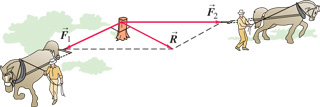Physics Practice Problems Vector Addition & Subtraction Practice Problems Solution: Two horses pull horizontally on ropes attached to ...

⚠️Our tutors found the solution shown to be helpful for the problem you're searching for. We don't have the exact solution yet.

# Solution: Two horses pull horizontally on ropes attached to a stump. The two forces exttip{vec {F_1}}{F_1_vec} = 1310 N and 2 that they apply to the stump are such that the net (resultant) force exttip{vec{R}}{R_vec} has a magnitude equal to that of 1 and makes an angle of 901 with 1. Find the magnitude of exttip{vec{F}_{ m 2}}{F_2_vec}.Find the direction of exttip{vec{F}_{ m 2}}{F_2_vec} (relative to exttip{vec {F_1}}{F_1_vec}).

###### Problem

Two horses pull horizontally on ropes attached to a stump. The two forces = 1310 N and 2 that they apply to the stump are such that the net (resultant) force has a magnitude equal to that of 1 and makes an angle of 901 with 1.Find the magnitude of .

Find the direction of (relative to ).

#### Q. While following a treasure map, you start at an old oak tree. You first walk 855 m directly south, then turn and walk 1.27 km at 30.0...

Solved • Mon Oct 29 2018 18:50:37 GMT-0400 (EDT)

#### Q. A disoriented physics professor drives 3.45 km north, then 2.45 km west, and then 1.50 km south.Find the magnitude of the r...

Solved • Mon Oct 29 2018 18:50:36 GMT-0400 (EDT)